# SLS机器学习最佳实践：时序异常检测

+关注继续查看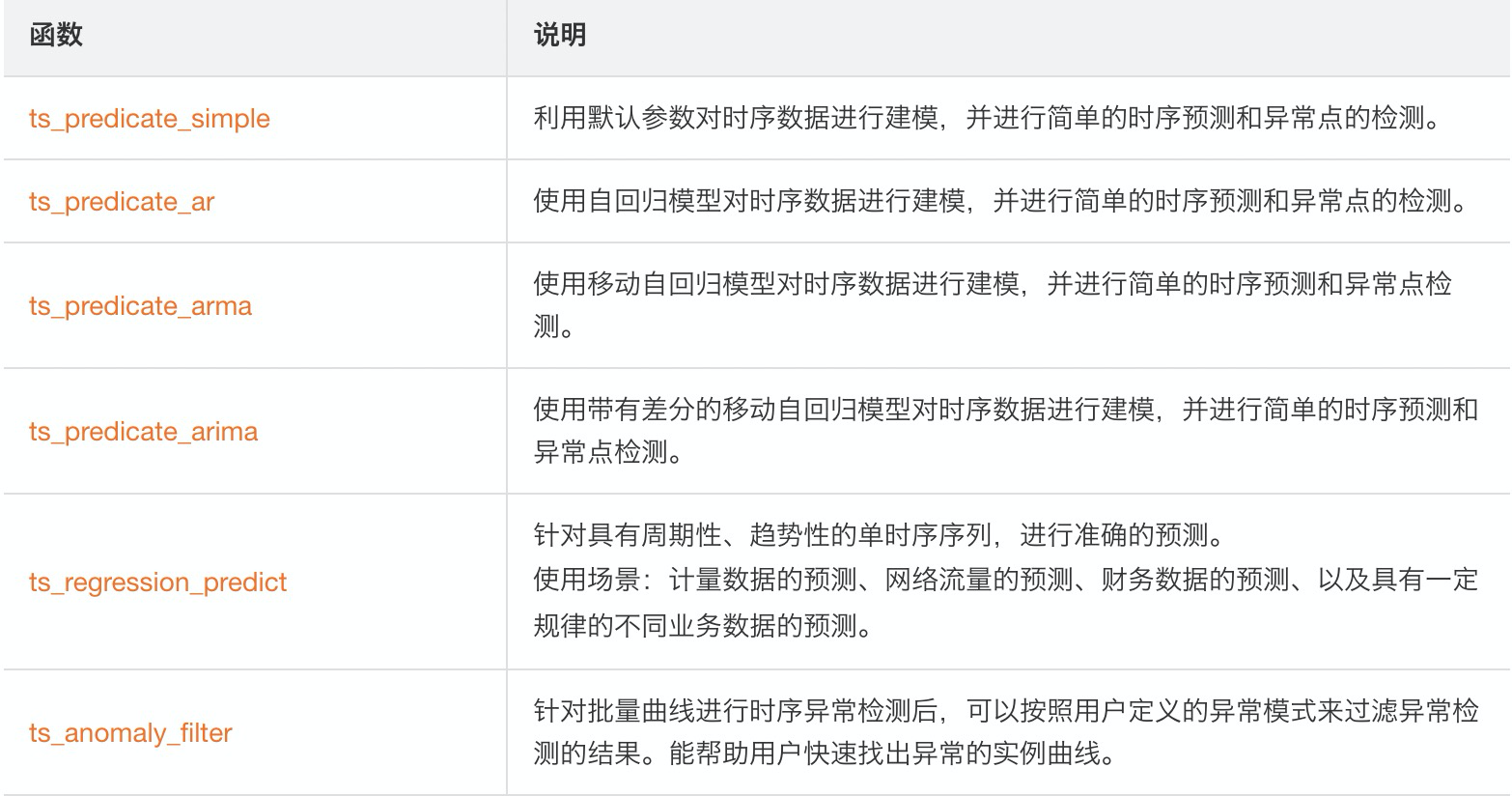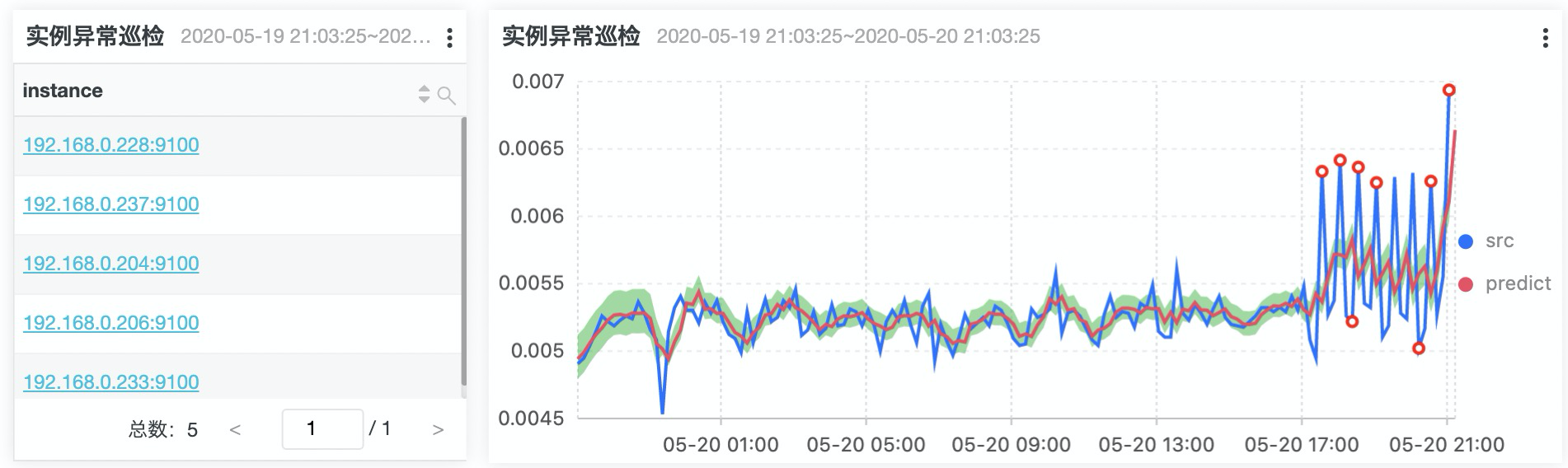• 最复杂的巡检SQL函数如下所示：
* |
SELECT res.name AS INSTANCE
FROM
(SELECT ts_anomaly_filter(INSTANCE, ts, ds, preds, probs, cast(5 AS bigint), cast(1 AS bigint)) AS res
FROM
(SELECT INSTANCE,
res AS ts,
res AS ds,
res AS preds,
res AS uppers,
res AS lowers,
res AS probs
FROM
(SELECT INSTANCE,
array_transpose(ts_predicate_arma(TIME, value, 5, 1, 1, 1, 1, TRUE)) AS res
FROM
(SELECT (TIME/1000) AS TIME,
labels['instance'] AS INSTANCE,
value
FROM
(SELECT promql_query_range('1 - avg(irate(node_cpu_seconds_total{instance=~".*",mode="idle"}[10m])) by (instance) ', '10m') AS t
FROM metrics)
ORDER BY TIME ASC)
GROUP BY INSTANCE)))

• 我们先获得到对应要检测的对象：
* |
SELECT (TIME/1000) AS TIME,
labels['instance'] AS INSTANCE,
value
FROM
(SELECT promql_query_range('1 - avg(irate(node_cpu_seconds_total{instance=~".*",mode="idle"}[10m])) by (instance) ', '10m') AS t
FROM metrics)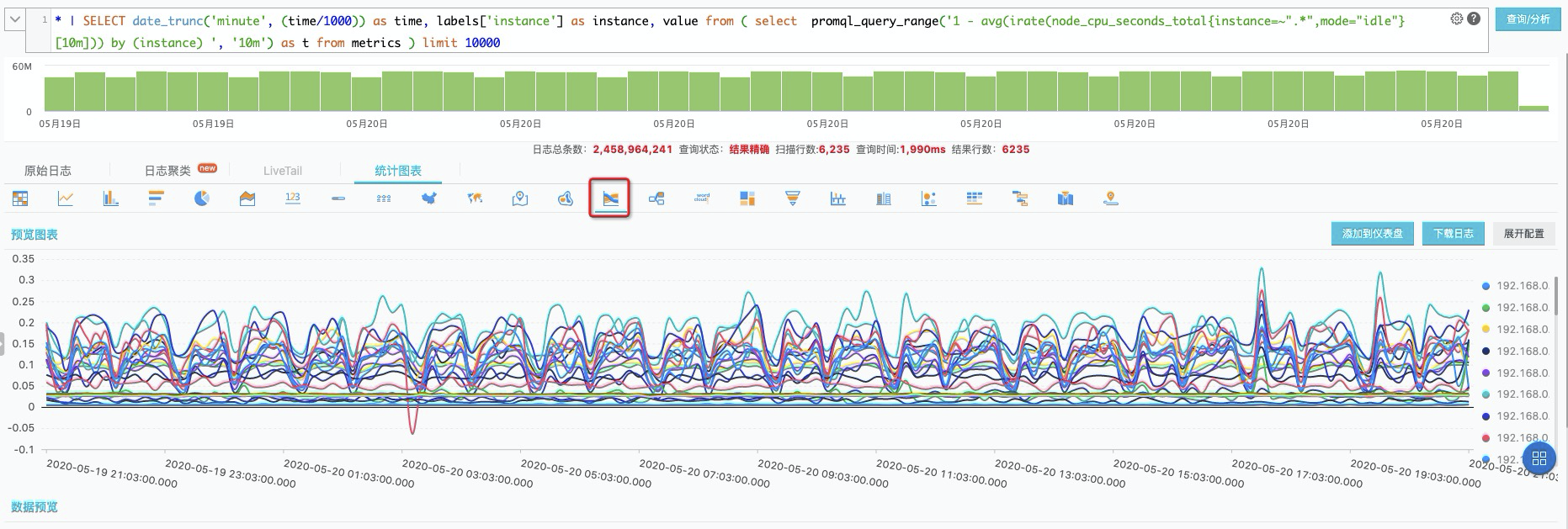• 我们要针对获取的N条线，进行异常检测。SLS提供了异常检测函数，同时支持group by模式，我们可以较为放方便的使用上述方法进行巡检
* |
SELECT INSTANCE,
ts_predicate_arma(TIME, value, 5, 1, 1, 1.0, 1.0, TRUE)
FROM
(SELECT (TIME/1000) AS TIME,
labels['instance'] AS INSTANCE,
value
FROM
(SELECT promql_query_range('1 - avg(irate(node_cpu_seconds_total{instance=~".*",mode="idle"}[10m])) by (instance) ', '10m') AS t
FROM metrics))
GROUP BY INSTANCE* |
SELECT INSTANCE,
array_transpose(ts_predicate_arma(TIME, value, 5, 1, 1, 1.0, 1.0, TRUE)) AS res
FROM
(SELECT (TIME/1000) AS TIME,
labels['instance'] AS INSTANCE,
value
FROM
(SELECT promql_query_range('1 - avg(irate(node_cpu_seconds_total{instance=~".*",mode="idle"}[10m])) by (instance) ', '10m') AS t
FROM metrics))
GROUP BY INSTANCE

* |
SELECT INSTANCE,
res AS ts,
res AS ds,
res AS preds,
res AS uppers,
res AS lowers,
res AS probs
FROM
(SELECT INSTANCE,
array_transpose(ts_predicate_arma(TIME, value, 5, 1, 1, 1.0, 1.0, TRUE)) AS res
FROM
(SELECT (TIME/1000) AS TIME,
labels['instance'] AS INSTANCE,
value
FROM
(SELECT promql_query_range('1 - avg(irate(node_cpu_seconds_total{instance=~".*",mode="idle"}[10m])) by (instance) ', '10m') AS t
FROM metrics))
GROUP BY INSTANCE)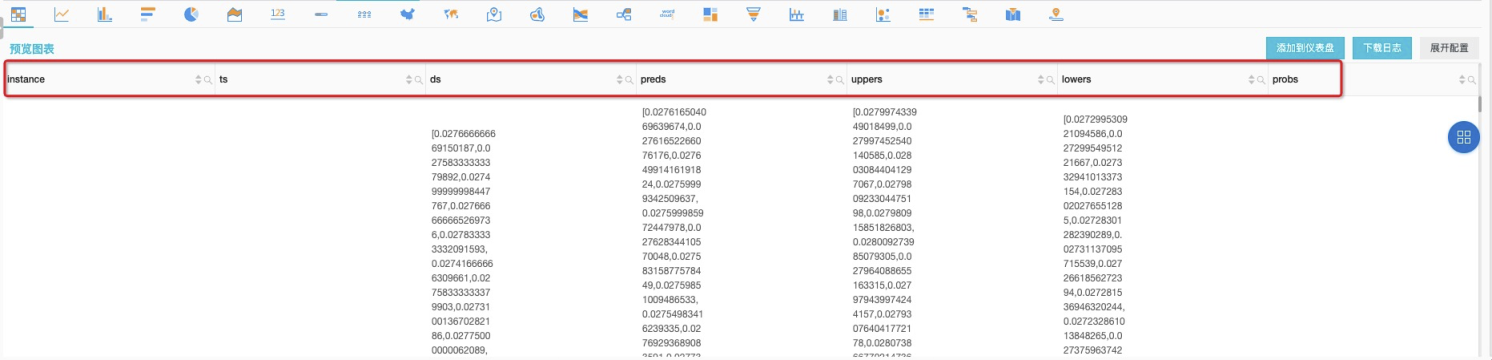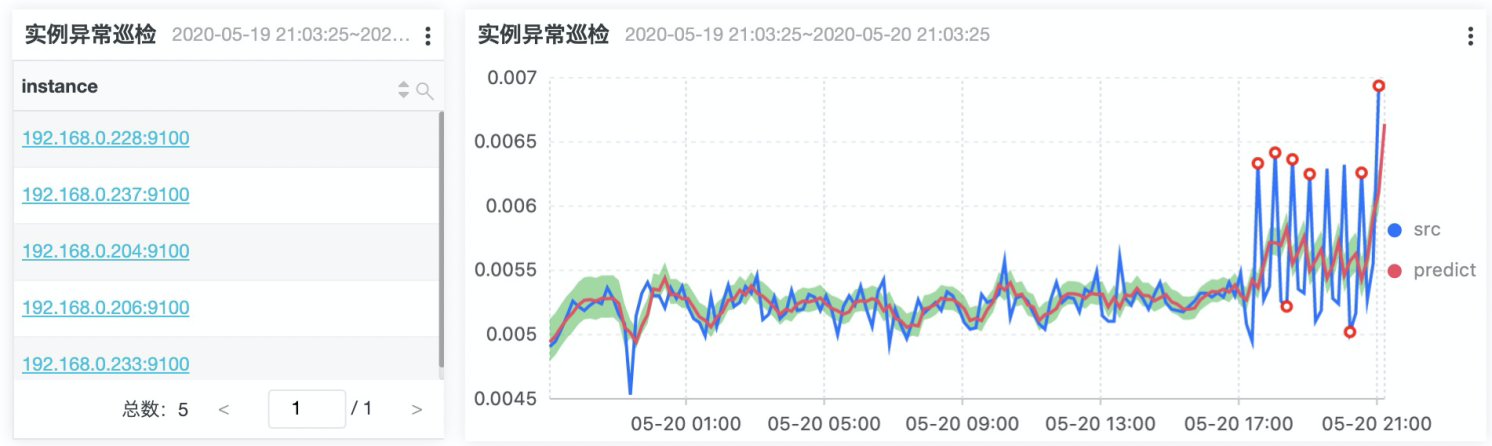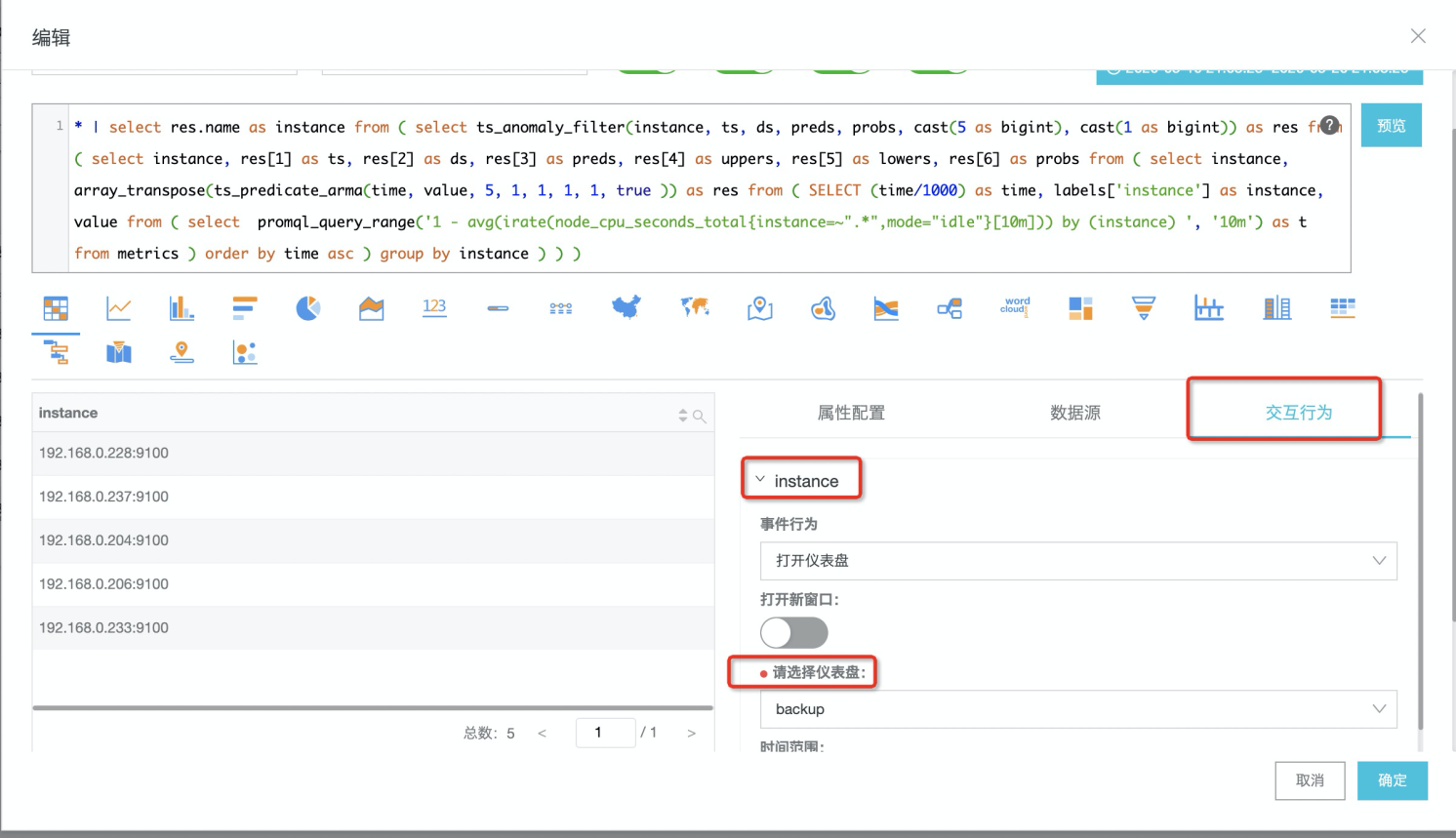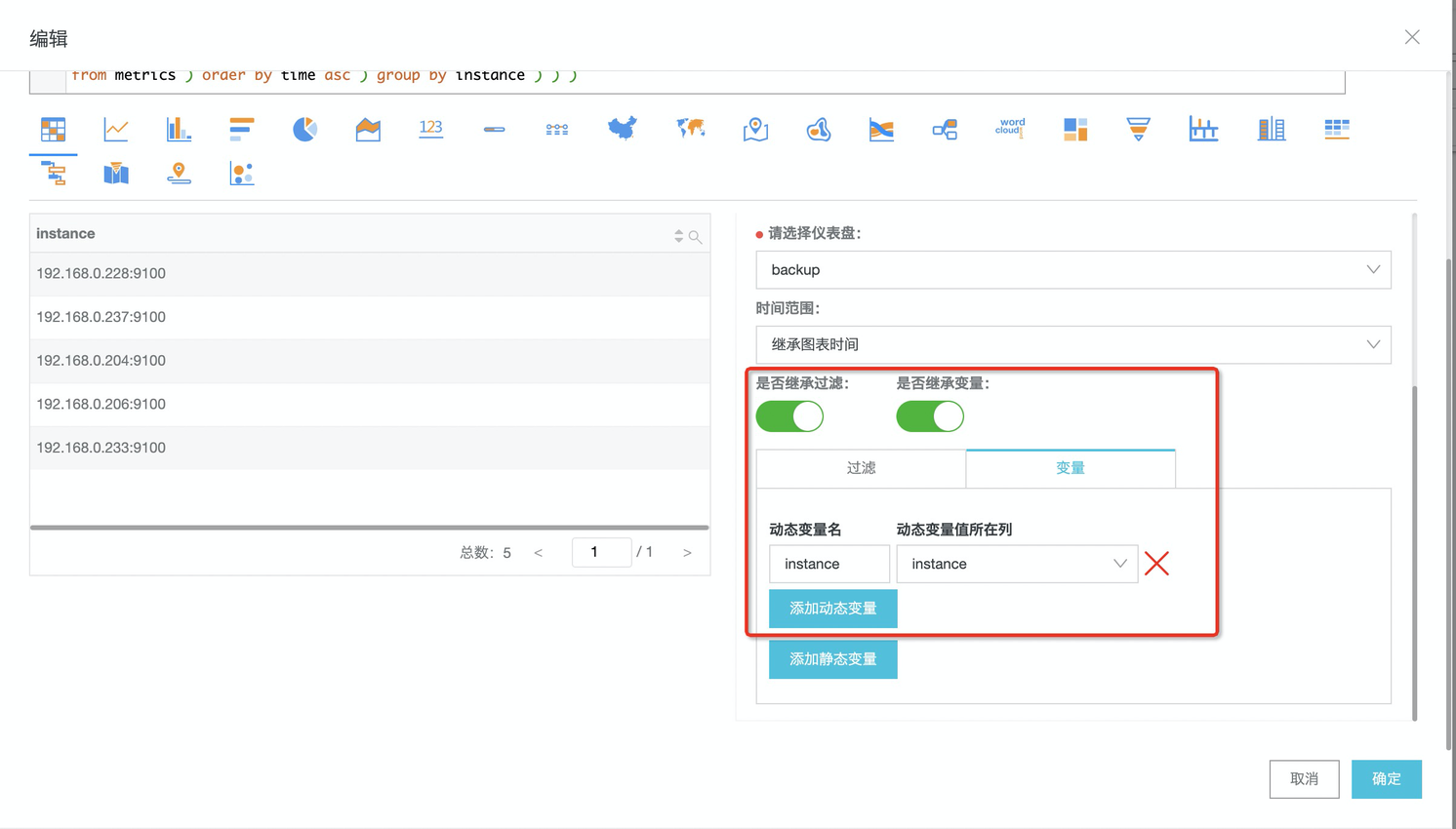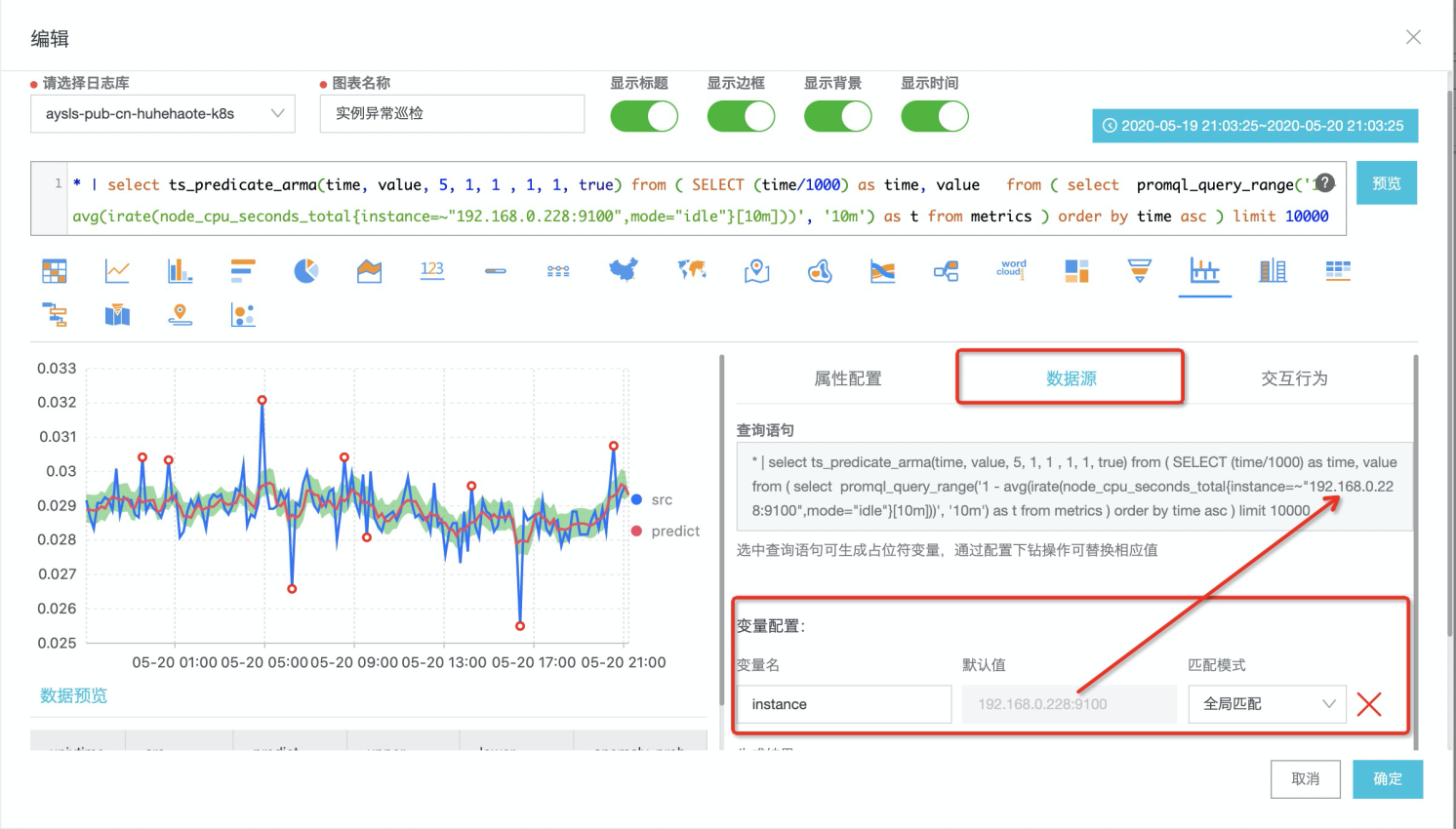《构建实时机器学习系统》一第2章 实时监督式机器学习 2.1　什么是监督式机器学习

1484 03130 02654 0877 07435 0[收藏学习]Linux的实时监测命令
1370 0《构建实时机器学习系统》一3.2　Pandas 的安装

1107 0《构建实时机器学习系统》一1.8　实时机器学习模型的生存期

1815 0《构建实时机器学习系统》一1.6　实时应用对机器学习的要求

947 0《数据科学：R语言实战》一1.2　异常检测
2152 0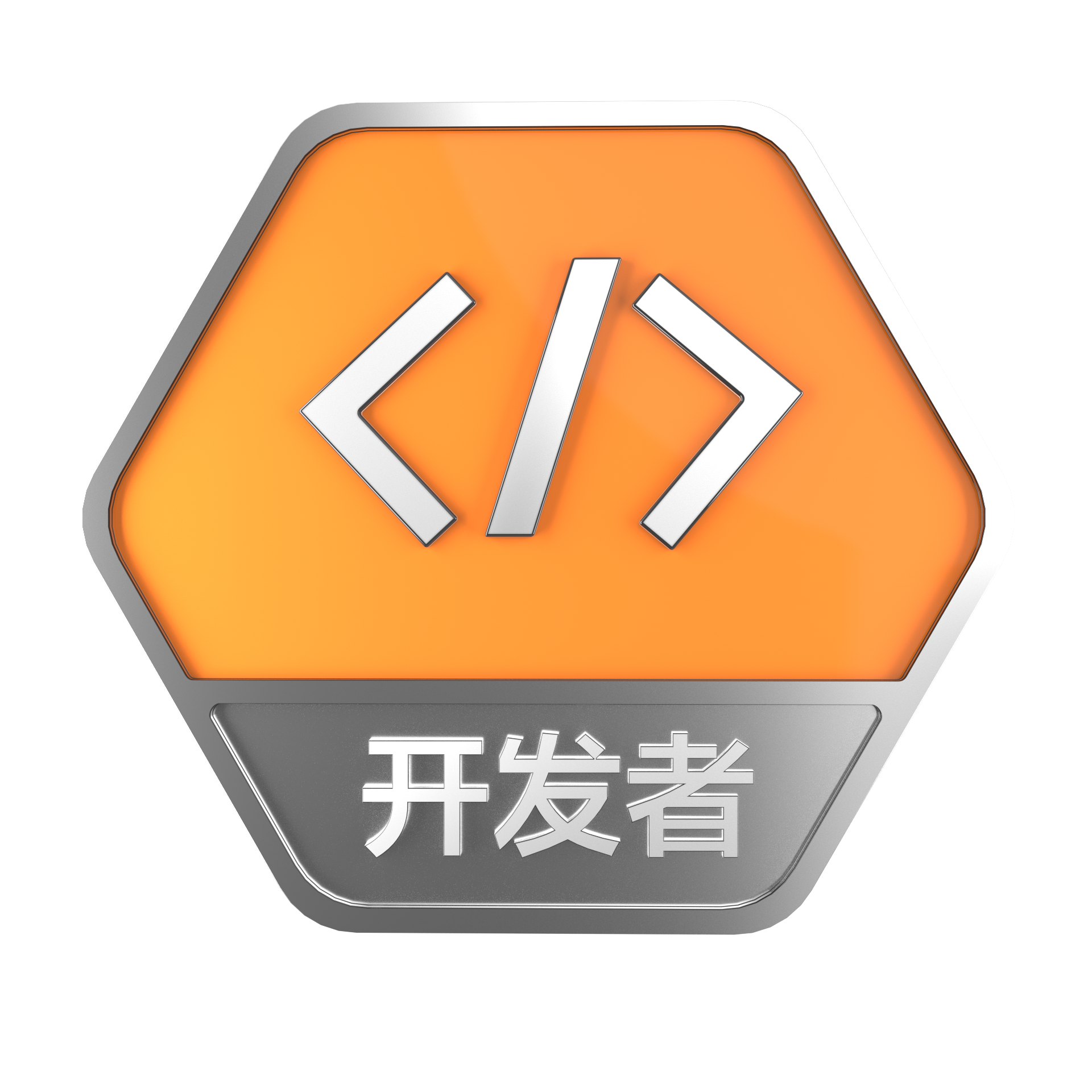+关注

22

1

+ 订阅

《SaaS模式云原生数据仓库应用场景实践》

《看见新力量：二》电子书